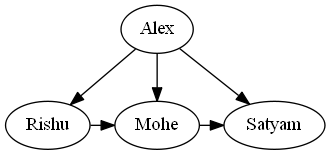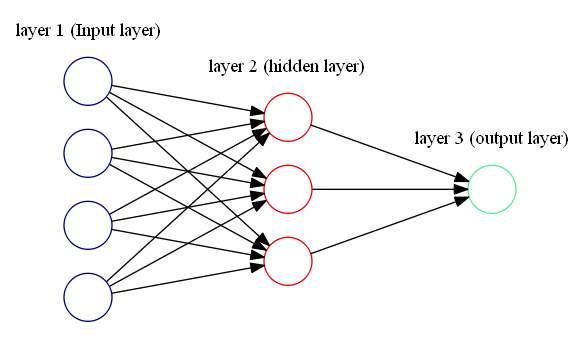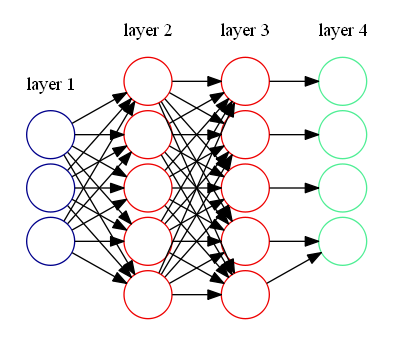GeeksforGeeks App
Open AppBrowser
Continue

# How to Visualize a Neural Network in Python using Graphviz ?

In this article, We are going to see how to plot (visualize) a neural network in python using Graphviz. Graphviz is a python module that open-source graph visualization software. It is widely popular among researchers to do visualizations. It’s representing structural information as diagrams of abstract graphs and networks means you only need to provide an only textual description of the graph regarding its topological structure and this will automatically read and create an image.

Installation:

For window terminal:

`pip install graphviz`

For anaconda terminal:

`conda install -c anaconda graphviz`

## Plotting a simple graph with Graphviz

Approach:

• Import module.
• Create a new object of Diagraph.
• Add node() and edge() into graph object.
• Save the source code with render() object.

Below is the implementation:

## Python3

 `# import module``from` `graphviz ``import` `Digraph`` ` `# instantiating object``dot ``=` `Digraph(comment``=``'A Round Graph'``)`` ` `# Adding nodes``dot.node(``'A'``, ``'Alex'``)``dot.node(``'B'``, ``'Rishu'``)``dot.node(``'C'``, ``'Mohe'``)``dot.node(``'D'``, ``'Satyam'``)`` ` `# Adding edges``dot.edges([``'AB'``, ``'AC'``, ``'AD'``])``dot.edge(``'B'``, ``'C'``, constraint ``=` `'false'``)``dot.edge(``'C'``, ``'D'``, constraint ``=` `'false'``)`` ` `# saving source code``dot.``format` `=` `'png'``dot.render(``'Graph'``, view ``=` `True``) `

Output:

`Graph.png`We can check the generated source code with dot.source methods:

## Python3

 `print``(dot.source)`

Output:

```// A Round Graph
digraph {
A [label=Alex]
B [label=Rishu]
C [label=Mohe]
D [label=Satyam]
A -> B
A -> C
A -> D
B -> C [constraint=false]
C -> D [constraint=false]
}```

## Plotting (visualize) a neural network with Graphviz

Here we are using source code for implementation which we see in the above examples:

Let’s discussed the approach:

• Create a digraph object.
• Define the direction of the graph using rankdir.
• Create a subgraph with the following things:
• Set color.
• Set node properties.
• Set Level of the subgraph
• Create the edge of between the object with (->).

This source code must be saved in a .txt file(myfile.txt) and run `dot -Tpng -O myfile.txt` from the command-line to get a .png figure with the diagram.

Example 1:

```digraph G {

rankdir=LR
splines=line

node [fixedsize=true, label=""];

subgraph cluster_0 {
color=white;
node [style=solid,color=blue4, shape=circle];
x1 x2 x3 x4;
label = "layer 1 (Input layer)";
}

subgraph cluster_1 {
color=white;
node [style=solid,color=red2, shape=circle];
a12 a22 a32;
label = "layer 2 (hidden layer)";
}

subgraph cluster_2 {
color=white;
node [style=solid,color=seagreen2, shape=circle];
O;
label="layer 3 (output layer)";
}

x1 -> a12;
x1 -> a22;
x1 -> a32;
x2 -> a12;
x2 -> a22;
x2 -> a32;
x3 -> a12;
x3 -> a22;
x3 -> a32;
x4 -> a12;
x4 -> a22;
x4 -> a32;

a12 -> O
a22 -> O
a32 -> O

}```

Run this into the terminal:

`dot -Tpng -O myfile.txt`

Output:Example 2:

```digraph G {

rankdir=LR
splines=line
nodesep=.05;

node [label=""];

subgraph cluster_0 {
color=white;
node [style=solid,color=blue4, shape=circle];
x1 x2 x3;
label = "layer 1";
}

subgraph cluster_1 {
color=white;
node [style=solid,color=red2, shape=circle];
a12 a22 a32 a42 a52;
label = "layer 2";
}

subgraph cluster_2 {
color=white;
node [style=solid,color=red2, shape=circle];
a13 a23 a33 a43 a53;
label = "layer 3";
}

subgraph cluster_3 {
color=white;
node [style=solid,color=seagreen2, shape=circle];
O1 O2 O3 O4;
label="layer 4";
}

x1 -> a12;
x1 -> a22;
x1 -> a32;
x1 -> a42;
x1 -> a52;

x2 -> a12;
x2 -> a22;
x2 -> a32;
x2 -> a42;
x2 -> a52;

x3 -> a12;
x3 -> a22;
x3 -> a32;
x3 -> a42;
x3 -> a52;

a12 -> a13
a22 -> a13
a32 -> a13
a42 -> a13
a52 -> a13

a12 -> a23
a22 -> a23
a32 -> a23
a42 -> a23
a52 -> a23

a12 -> a33
a22 -> a33
a32 -> a33
a42 -> a33
a52 -> a33

a12 -> a43
a22 -> a43
a32 -> a43
a42 -> a43
a52 -> a43

a12 -> a53
a22 -> a53
a32 -> a53
a42 -> a53
a52 -> a53

a13 -> O1
a23 -> O2
a33 -> O3
a43 -> O4
a53 -> O4

}```

Output:My Personal Notes arrow_drop_up